# JP2018203034A - Travel track determination device and automatic driving device - Google Patents

## Info

Publication number
JP2018203034A
JP2018203034A JP2017110415A JP2017110415A JP2018203034A JP 2018203034 A JP2018203034 A JP 2018203034A JP 2017110415 A JP2017110415 A JP 2017110415A JP 2017110415 A JP2017110415 A JP 2017110415A JP 2018203034 A JP2018203034 A JP 2018203034A
Authority
JP
Japan
Prior art keywords
travel
value
trajectory
future
traveling
Prior art date
Legal status (The legal status is an assumption and is not a legal conclusion. Google has not performed a legal analysis and makes no representation as to the accuracy of the status listed.)
Granted
Application number
JP2017110415A
Other languages
Japanese (ja)
Other versions
JP6580087B2 (en
Inventor

Yuji Yasui

Original Assignee

Honda Motor Co Ltd
Priority date (The priority date is an assumption and is not a legal conclusion. Google has not performed a legal analysis and makes no representation as to the accuracy of the date listed.)
Filing date
Publication date
Application filed by 本田技研工業株式会社, Honda Motor Co Ltd filed Critical 本田技研工業株式会社
Priority to JP2017110415A priority Critical patent/JP6580087B2/en
Publication of JP2018203034A publication Critical patent/JP2018203034A/en
Application granted granted Critical
Publication of JP6580087B2 publication Critical patent/JP6580087B2/en
Application status is Active legal-status Critical
Anticipated expiration legal-status Critical

• 238000004364 calculation methods Methods 0 claims description 151
• 238000004422 calculation algorithm Methods 0 claims description 29
• 238000000034 methods Methods 0 description 20
• 238000004088 simulation Methods 0 description 8

## Images

•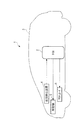•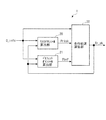•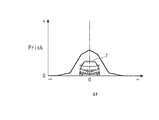•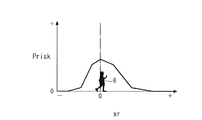••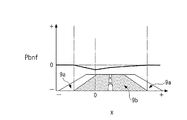•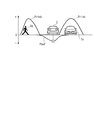•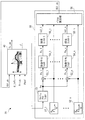•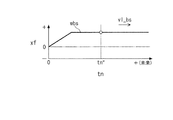•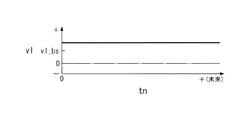•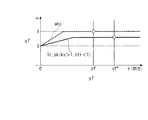•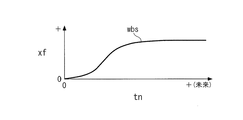•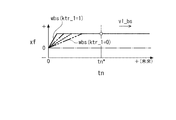•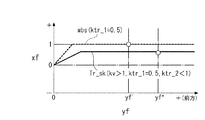•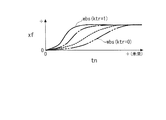••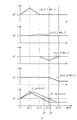•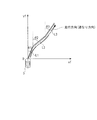•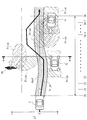•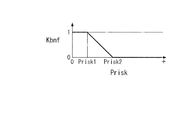•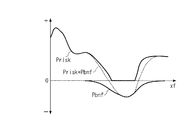•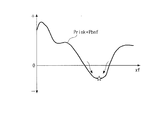•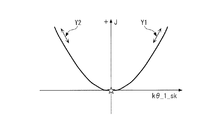•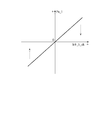•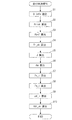••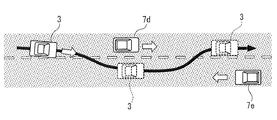•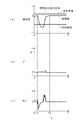•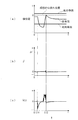•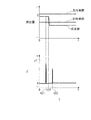## Classifications

• BPERFORMING OPERATIONS; TRANSPORTING
• B60VEHICLES IN GENERAL
• B60WCONJOINT CONTROL OF VEHICLE SUB-UNITS OF DIFFERENT TYPE OR DIFFERENT FUNCTION; CONTROL SYSTEMS SPECIALLY ADAPTED FOR HYBRID VEHICLES; ROAD VEHICLE DRIVE CONTROL SYSTEMS FOR PURPOSES NOT RELATED TO THE CONTROL OF A PARTICULAR SUB-UNIT
• B60W30/00Purposes of road vehicle drive control systems not related to the control of a particular sub-unit, e.g. of systems using conjoint control of vehicle sub-units, or advanced driver assistance systems for ensuring comfort, stability and safety or drive control systems for propelling or retarding the vehicle
• GPHYSICS
• G05CONTROLLING; REGULATING
• G05DSYSTEMS FOR CONTROLLING OR REGULATING NON-ELECTRIC VARIABLES
• G05D1/00Control of position, course or altitude of land, water, air, or space vehicles, e.g. automatic pilot
• G05D1/02Control of position or course in two dimensions
• G05D1/021Control of position or course in two dimensions specially adapted to land vehicles
• G05D1/0212Control of position or course in two dimensions specially adapted to land vehicles with means for defining a desired trajectory
• G05D1/0214Control of position or course in two dimensions specially adapted to land vehicles with means for defining a desired trajectory in accordance with safety or protection criteria, e.g. avoiding hazardous areas
• BPERFORMING OPERATIONS; TRANSPORTING
• B60VEHICLES IN GENERAL
• B60WCONJOINT CONTROL OF VEHICLE SUB-UNITS OF DIFFERENT TYPE OR DIFFERENT FUNCTION; CONTROL SYSTEMS SPECIALLY ADAPTED FOR HYBRID VEHICLES; ROAD VEHICLE DRIVE CONTROL SYSTEMS FOR PURPOSES NOT RELATED TO THE CONTROL OF A PARTICULAR SUB-UNIT
• B60W40/00Estimation or calculation of non-directly measurable driving parameters for road vehicle drive control systems not related to the control of a particular sub unit, e.g. by using mathematical models
• B60W40/02Estimation or calculation of non-directly measurable driving parameters for road vehicle drive control systems not related to the control of a particular sub unit, e.g. by using mathematical models related to ambient conditions
• GPHYSICS
• G05CONTROLLING; REGULATING
• G05DSYSTEMS FOR CONTROLLING OR REGULATING NON-ELECTRIC VARIABLES
• G05D1/00Control of position, course or altitude of land, water, air, or space vehicles, e.g. automatic pilot
• G05D1/0088Control of position, course or altitude of land, water, air, or space vehicles, e.g. automatic pilot characterized by the autonomous decision making process, e.g. artificial intelligence, predefined behaviours
• BPERFORMING OPERATIONS; TRANSPORTING
• B60VEHICLES IN GENERAL
• B60WCONJOINT CONTROL OF VEHICLE SUB-UNITS OF DIFFERENT TYPE OR DIFFERENT FUNCTION; CONTROL SYSTEMS SPECIALLY ADAPTED FOR HYBRID VEHICLES; ROAD VEHICLE DRIVE CONTROL SYSTEMS FOR PURPOSES NOT RELATED TO THE CONTROL OF A PARTICULAR SUB-UNIT
• B60W2554/00Input parameters relating to objects
• BPERFORMING OPERATIONS; TRANSPORTING
• B60VEHICLES IN GENERAL
• B60WCONJOINT CONTROL OF VEHICLE SUB-UNITS OF DIFFERENT TYPE OR DIFFERENT FUNCTION; CONTROL SYSTEMS SPECIALLY ADAPTED FOR HYBRID VEHICLES; ROAD VEHICLE DRIVE CONTROL SYSTEMS FOR PURPOSES NOT RELATED TO THE CONTROL OF A PARTICULAR SUB-UNIT
• B60W2556/00Input parameters relating to data
• B60W2556/45External transmission of data to or from the vehicle
• B60W2556/50External transmission of data to or from the vehicle for navigation systems
• GPHYSICS
• G05CONTROLLING; REGULATING
• G05DSYSTEMS FOR CONTROLLING OR REGULATING NON-ELECTRIC VARIABLES
• G05D2201/00Application
• G05D2201/02Control of position of land vehicles
• G05D2201/0212Driverless passenger transport vehicle

## Abstract

A traveling track determination device capable of quickly and appropriately determining a future traveling track of a host vehicle and an automatic driving device including the same are provided.
A traveling track determination apparatus includes an ECU. The ECU 2 calculates a risk potential Prisk representing a region where the traffic participant may exist in the future from the present in the vicinity of the host vehicle 3 according to the surrounding situation data D_info detected by the situation detecting device 5. A benefit potential Pbnf representing an ideal future travel region in which the host vehicle 3 should travel is calculated, and a future travel trajectory Tr_sk of the host vehicle 3 is determined using the risk potential Prisk and the benefit potential Pbnf.
[Selection] Figure 19

## Description

The present invention relates to a traveling track determination device and an automatic driving device that determine a future traveling track of a host vehicle.

As a traveling track determination device that determines the future traveling track of the host vehicle, a device described in Patent Document 1 is known. This traveling trajectory determination device is applied to a mobile robot control device that controls a mobile robot vehicle (hereinafter referred to as “own vehicle”). This mobile robot control device stores map information including a travel rule, an external sensor for measuring distance data between the host vehicle and an obstacle in the moving direction, an antenna for receiving position information from GPS and the like. Storage unit, obstacle map creation unit and the like. The obstacle map creation unit creates an obstacle map based on the distance data measured by the external sensor.

In this travel trajectory determination device, a future travel trajectory from the current position to the target position is determined by the algorithm shown in FIG. That is, the current position of the host vehicle is calculated based on the movement amount of the host vehicle, the obstacle map is created based on the distance data measured by the external sensor, and the map information is read from the storage unit (step 201 to step 201). 203).

Next, referring to the obstacle map and the map information, it is determined whether the obstacle exists on the trajectory stored in the map information (step 204). If the obstacle exists on the trajectory, The travel trajectory search is executed by the A * search algorithm (step 205). Specifically, based on the current position information, obstacle map, and map information, the obstacle existence probability (route search cost) in many grids surrounding the vehicle on the grid map is calculated, and the obstacle existence probability The grid with the lowest is determined / selected as a future trajectory. And the control command signal value for making the own vehicle drive | work on the determined driving | running track is calculated, and the control command signal equivalent to it is input into the drive part of the own vehicle (steps 206-207).

JP 2010-191502 A

According to the traveling track determination device of Patent Document 1, the existence probability of obstacles in a large number of grids surrounding the host vehicle must be calculated based on the current position information, the obstacle map, and the map information in one calculation cycle. Therefore, the calculation of the arithmetic unit becomes heavy. As a result, the manufacturing cost of the arithmetic device is increased, and the calculation capability may be difficult with the capability of the arithmetic device currently installed in the automobile.

Further, for the same reason, the calculation time of the calculation device becomes long, and there is a possibility that the calculation will not be completed in one calculation cycle. In this case, the traveling track is not determined, In order for the vehicle to stop running or to secure the calculation time, the host vehicle can only run at a low speed. As a result, it cannot be applied to a vehicle that requires high-speed driving such as an autonomous driving vehicle that travels on a general road or a highway, resulting in a reduction in merchantability.

Furthermore, under the condition that there are many grids with the lowest probability of obstacles, there is a problem that it is not possible to select an optimal traveling track that is smoother and safer. In this case, it becomes more remarkable. In addition, the above problem occurs similarly when the CC-RRT method is used instead of the A * search algorithm in the search for the traveling track.

The present invention has been made to solve the above-described problems, and provides a traveling track determination device that can quickly and appropriately determine the future traveling track of the host vehicle and an automatic driving device including the traveling track determination device. Objective.

In order to achieve the above object, the traveling track determining apparatus 1 according to claim 1 includes a surrounding situation data acquisition means (situation detecting apparatus 5) for obtaining surrounding situation data D_info representing the surrounding situation of the host vehicle 3, and the surrounding situation. Using the data D_info, there is a possibility that traffic participants (other vehicles 77a to 7e, pedestrians 8, 8a, and 8b) around the host vehicle 3 may exist in the future from the present or in the future. Existing region data calculating means (ECU2, risk potential calculating unit 20) for calculating existing region data (risk potential Risk) representing an existing region that is a certain region, existing region data (risk potential Risk) and a predetermined function value ( Reference travel trajectory wbs, trajectory weight function value Wtr_i, reference road direction speed v_bs) are corrected values (vertical speed A travel trajectory determining means for determining the future travel trajectory Tr_sk of the host vehicle 3 using the values corrected by the positive coefficient kv, the trajectory correction coefficients ktr, ktr_2, ktr2_i, the speed correction coefficient kv_i, and the search trajectory correction vector Kθ). Using the ECU 2 and the travel environment model estimation unit 40) and a predetermined algorithm (Equations (1) to (30)), the degree of intersection between the future travel trajectory Tr_sk and the existence area represented by the existence area data includes a minimum value. Correction value calculation means (ECU2, extreme value search controller 50) for calculating a correction value (search trajectory correction vector Kθ) so as to be a value in the vicinity of the minimum value.

According to this traveling track determination device, the surrounding situation data representing the surrounding situation of the own vehicle is acquired, and using this surrounding situation data, there is a possibility that traffic participants around the own vehicle may exist from the present to the future. Presence area data representing an existence area that may exist in a certain area or in the future is calculated, and using the existence area data and a value obtained by correcting a predetermined function value with a correction value, A traveling track is determined. This correction value is calculated using a predetermined algorithm so that the degree of intersection between the future traveling track and the existence area represented by the existence area data becomes a value near the minimum value including the minimum value. When calculating the future travel trajectory of the vehicle, unlike the case of Patent Document 1, it is not necessary to calculate / estimate the existence probability of obstacles in a large number of grids surrounding the host vehicle. Can be determined promptly and appropriately while avoiding crossing with the presence area of the traffic participant. As a result, when this traveling track determination device is applied to an autonomous driving vehicle, a safe and ideal traveling state can be realized by automatic driving as in the case of driving by a skilled driver. (In this specification, “acquisition” of “acquisition of surrounding situation data” is not limited to directly detecting the surrounding situation data by a sensor or the like, and the surrounding situation data is determined based on other parameters. In addition, “transport participants” in this specification includes pedestrians, other vehicles, and obstacles).

According to a second aspect of the present invention, in the traveling track determining apparatus 1 according to the first aspect, the predetermined function value (reference traveling path wbs) is a function that predefines the form when the host vehicle 3 changes course as a map. It is a value.

According to this travel track determination device, the future travel track of the host vehicle is determined using a value obtained by correcting the function value defined in advance as a map with the form when the host vehicle changes course, It is possible to avoid the future traveling track of the host vehicle from being determined to be a future traveling track that the host vehicle cannot follow while avoiding the intersection of the future traveling track of the host vehicle with the area where the traffic participant exists. As a result, when this traveling track determination device is applied to an autonomous driving vehicle, it realizes a safe and ideal traveling state by automatic driving while preventing the vehicle from exhibiting unstable behavior such as spin and meandering. can do.

According to a third aspect of the present invention, in the traveling track determining apparatus 1 according to the second aspect, the predetermined function value (reference traveling path wbs) is a function value defining a course changing speed when the host vehicle 3 changes the course. The correction values (longitudinal speed correction coefficient kv, trajectory correction coefficients ktr, ktr_2) are configured to correct the course changing speed.

According to this traveling track determination device, the predetermined function value is a function value that defines the course changing speed when the host vehicle changes course, and the correction value is configured to correct the course changing speed. The speed in the lateral direction of the host vehicle can be set appropriately. Thereby, when this traveling track determination device is applied to an autonomous driving vehicle, smooth automatic driving can be realized.

According to a fourth aspect of the present invention, in the traveling trajectory determination device 1 according to the first aspect, the predetermined function value is composed of a plurality of function values that intersect each other, and the correction value includes a plurality of correction values (track correction coefficients). ktr2_i), and the traveling trajectory determining means combines a plurality of values obtained by correcting a plurality of function values (trajectory weighting function values Wtr_i) with a plurality of correction values (orbit correction coefficient ktr2_i), respectively. The trajectory Tr_sk is determined.

According to this traveling trajectory determination device, a predetermined function value is composed of a plurality of function values intersecting each other, the correction value is composed of a plurality of correction values, and the plurality of function values are composed of a plurality of correction values. Combining multiple corrected values will determine the future travel trajectory, so that the vehicle will move to the left while avoiding the future travel trajectory of the vehicle crossing the area where the traffic participant exists. After changing the course, it is possible to appropriately set the future traveling trajectory under conditions such as changing the course to the right side or repeating zigzag traveling. As a result, when this traveling track determination device is applied to an autonomous driving vehicle, smooth and safe automatic operation is possible when the vehicle changes its course to the left and then changes to the right or repeats zigzag traveling. Driving can be realized.

The invention according to claim 5 is the traveling trajectory determination device 1 according to claim 1, wherein the predetermined function value is composed of a function value obtained by combining a plurality of line segments with an angle between each other, and is corrected. The value (speed correction coefficient kv_i) is configured to correct at least one of the length and angle of a plurality of line segments.

According to this traveling trajectory determination device, the predetermined function value is composed of a function value obtained by combining a plurality of line segments with an angle between each other, and the correction value is at least of the length and angle of the plurality of line segments. Since it is configured to correct one side, the vehicle's future traveling trajectory avoids crossing with the traffic participant's existing area, while the vehicle turns left or right at the intersection, or on the expressway It is possible to appropriately set the future traveling trajectory under conditions such as when joining. As a result, when this traveling track determination device is applied to an autonomous driving vehicle, smooth and safe automatic driving under conditions such as when the vehicle turns left or right at an intersection or merges on a highway. Can be realized.

According to a sixth aspect of the present invention, in the traveling track determining apparatus 1 according to any one of the first to fifth aspects, the correction value calculating means uses a predetermined algorithm (Equations (1) to (30)) to determine the future. While calculating the direction value (moving average value Pa_i) indicating the direction of change in the degree of intersection between the future traveling trajectory Tr_sk and the existing region represented by the existing region data when the traveling trajectory Tr_sk is changed, the direction value (moving average value Pa_i) is calculated. Using the average value Pa_i), a correction value (search trajectory correction vector Kθ) is calculated so that the future traveling trajectory Tr_sk is changed in a direction in which the degree of intersection with the existing region decreases.

According to this traveling trajectory determination device, using a predetermined algorithm, a direction value representing the direction of change in the degree of intersection between the future traveling trajectory and the existing area represented by the existing area data when the future traveling trajectory is changed. Is calculated, and the correction value is calculated using the direction value so that the future travel trajectory is changed in a direction in which the degree of intersection with the existing area decreases, so when calculating the future travel trajectory Calculation time can be shortened and calculation load can be reduced. As a result, the manufacturing cost of the traveling track determination device can be reduced, and the traveling track determination device can be realized by using a relatively low-performance computing device. For the above reasons, when this traveling track determination device is applied to an autonomous driving vehicle, the autonomous driving vehicle can be driven at high speed.

According to a seventh aspect of the present invention, in the travel path determining device 1 according to any one of the first to sixth aspects, a travel impossible area representing a travel impossible area where the host vehicle 3 should not travel using the surrounding situation data D_info. The vehicle further includes a non-running region data calculating unit (ECU2, risk potential calculating unit 20) for calculating data (risk potential Risk), and the correction value calculating unit uses a predetermined algorithm (Equations (1) to (30)). In addition to the degree of intersection between the future travel trajectory Tr_sk and the existence area (risk potential Prisk) represented by the existence area data, the intersection between the future travel trajectory Tr_sk and the travel impossible area (risk potential Prisk) represented by the impossible travel area data. The correction value (search trajectory correction vector) so that the degree becomes a value near the minimum value including the minimum value. And calculating a theta).

According to this travel trajectory determination device, the travel impossible area data representing the travel impossible area where the host vehicle should not travel is calculated using the surrounding situation data, and the future travel trajectory and the existence area are calculated using a predetermined algorithm. In addition to the degree of intersection with the existence area represented by the data, the correction value is set so that the degree of intersection between the future traveling track and the non-driving area represented by the non-driving area data becomes a value around the minimum value including the minimum value. Since it is calculated, it is possible to avoid the future traveling trajectory of the host vehicle from intersecting with the untravelable region. Thereby, when this traveling track determination device is applied to an autonomous driving vehicle, an ideal traveling state can be realized by automatic driving while ensuring higher safety.

According to an eighth aspect of the present invention, in the travel trajectory determining device 1 according to any one of the first to sixth aspects, travel region data (benefits potential Pbnf) representing an ideal future travel region in which the vehicle 3 should travel. Travel region data calculation means (ECU2, benefit potential calculation unit 21) for calculating the future travel trajectory Tr_sk using a predetermined algorithm (Equations (1) to (30)). The degree of intersection with the existence area represented by the existence area data becomes a value near the minimum value including the minimum value, and the degree of intersection between the future traveling trajectory Tr_sk and the traveling area represented by the traveling area data includes the maximum value. The correction value (search trajectory correction vector Kθ) is calculated so as to be a value near the value.

According to this traveling track determination device, traveling region data representing an ideal future traveling region in which the host vehicle should travel is calculated, and using a predetermined algorithm, a future traveling track and an existing region represented by existing region data. So that the degree of intersection between the future running track and the running area represented by the running area data becomes a value around the maximum value including the maximum value. Since the correction value is calculated, the future travel trajectory of the own vehicle is avoided quickly and appropriately so as to intersect with the ideal future travel area as much as possible while avoiding the intersection with the area where the traffic participant exists. Can be determined.

According to a ninth aspect of the present invention, in the travel trajectory determining device 1 according to the eighth aspect, when the correction value means intersects the travel area represented by the travel area data and the existence area represented by the existence area data. The correction value (search trajectory correction vector Kθ) is calculated so that the degree of intersection between the future travel trajectory Tr_sk and the existing area represented by the existing area data decreases as the existence probability of the traffic participant in the intersected area increases. It is characterized by

According to this traveling track determination device, when the traveling area represented by the traveling area data and the existence area represented by the existence area data are interlaced, the higher the probability that a traffic participant exists in the intersecting area, the more the future Since the correction value is calculated so that the degree of intersection between the running trajectory and the existence area represented by the existence area data is further reduced, under the condition where the ideal future driving area and the existence area of the traffic participant intersect It is possible to quickly and appropriately determine the future traveling track of the host vehicle while reducing the probability that a traffic participant exists on the traveling track.

The traveling track determination device 1 according to claim 10 uses the surrounding situation data acquisition means (situation detection device 5) for obtaining surrounding situation data D_info representing the surrounding situation of the own vehicle 3, and the surrounding situation data D_info, In the vicinity of 3, presence of traffic participants (other vehicles 7, 7 a to 7 e, pedestrians 8, 8 a, 8 b) that may exist in the future from the present or an area that may exist in the future Existence area data calculation means (ECU2, risk potential calculation unit 20) for calculating existence area data (risk potential Risk) representing an area, and travel area data (ideal future travel area where the host vehicle 3 should travel) ( Traveling area data calculation means (ECU2, benefit potential calculation unit 21) for calculating the benefit potential Pbnf) Traveling track determination means (ECU 2, traveling environment model estimation unit 40) for determining the future traveling track Tr_sk of the host vehicle 3 using the existence region data (risk potential Prisk) and the traveling region data (benefit potential Pbnf); It is characterized by providing.

According to this traveling track determination device, the surrounding situation data representing the surrounding situation of the own vehicle is acquired, and there is a possibility that traffic participants around the own vehicle exist from the present to the future using the surrounding situation data. Existence area data that represents an area or an area that may exist in the future is calculated, and travel area data that represents an ideal future travel area in which the host vehicle should travel is calculated. And since the future traveling track of the own vehicle is determined using the existence region data and the traveling region data, unlike the case of Patent Document 1, the future traveling track of the own vehicle is surrounded. By eliminating the need to calculate / estimate the existence probability of obstacles in many grids, the future driving trajectory of the host vehicle is prevented from intermingling with the area where the traffic participant exists, and the future driving of the host vehicle is avoided. The trajectory can be determined quickly and appropriately. As a result, when this traveling track determination device is applied to an autonomous driving vehicle, a safe and ideal traveling state can be realized by automatic driving as in the case of driving by a skilled driver, and at a high speed traveling state Can be realized by automatic operation. Thereby, high merchantability can be ensured.

According to an eleventh aspect of the present invention, in the traveling trajectory determining apparatus 1 according to the tenth aspect, the traveling trajectory determining means has a degree of intersection between the future traveling trajectory Tr_sk and the existence area represented by the existence area data (risk potential Risk). The value is in the vicinity of the minimum value including the minimum value, and the degree of intersection between the future travel trajectory Tr_sk and the travel region represented by the travel region data (benefit potential Pbnf) is a value in the vicinity of the maximum value including the maximum value. And determining a future travel track Tr_sk.

According to this travel trajectory determination device, the degree of intersection between the future travel trajectory and the existence region represented by the existence region data becomes a value near the minimum value including the minimum value, and the future travel trajectory and the travel region data represent Since the future travel trajectory is determined so that the degree of intersection with the travel area becomes a value near the maximum value including the maximum value, the future travel trajectory of the own vehicle intersects with the existence area of the traffic participant. It is possible to determine quickly and appropriately so as to intersect with the ideal future driving area as much as possible. As a result, when this traveling track determination device is applied to an autonomous driving vehicle, a safe and ideal traveling state can be realized by automatic driving as in the case of driving by a skilled driver. Can be secured.

According to a twelfth aspect of the present invention, in the traveling trajectory determining device 1 according to the tenth or eleventh aspect, the traveling trajectory determining means determines a reference traveling trajectory (reference trajectory wbs) that serves as a reference for the future traveling trajectory Tr_sk, The reference travel trajectory (reference trajectory wbs) becomes a value near the minimum value including the minimum value in the degree of intersection between the future travel trajectory Tr_sk and the region represented by the existing region data, and the future travel trajectory Tr_sk and the travel region data. The future travel trajectory Tr_sk is determined by correcting the degree of intersection with the travel area represented by the formula (1) so as to be a value in the vicinity of the maximum value including the maximum value.

According to this travel trajectory determination device, a reference travel trajectory serving as a reference for the future travel trajectory is determined, and the degree of intersection between the future travel trajectory and the region represented by the existing region data includes a minimum value. By correcting the value so that the intersection between the future travel path and the travel area represented by the travel area data becomes a value near the maximum value including the maximum value, the future travel path becomes a value near the minimum value. Therefore, it is possible to quickly and appropriately determine the future travel trajectory so as to intersect with the ideal future travel area as much as possible while avoiding the intersection with the area where the traffic participant exists. it can.

According to a thirteenth aspect of the present invention, in the traveling trajectory determining device 1 according to the eleventh aspect, the traveling trajectory determining means is configured such that the traveling area represented by the traveling area data intersects with the existing area represented by the existing area data. Is to determine the future traveling trajectory Tr_sk such that the higher the presence probability of the traffic participant in the intersecting area, the more the degree of intersection between the future traveling trajectory Tr_sk and the existing area represented by the existing area data decreases. Features.

According to this traveling track determination device, when the traveling area represented by the traveling area data and the existence area represented by the existence area data are interlaced, the higher the probability that a traffic participant exists in the intersecting area, the more the future Since the future driving trajectory is determined so that the degree of intersection between the driving trajectory and the existing area represented by the existing area data is further reduced, the ideal future driving area and the traffic participant existing area intersect Therefore, it is possible to quickly and appropriately determine the future traveling track of the host vehicle while reducing the probability that a traffic participant exists on the traveling track.

An automatic driving device 1 according to a fourteenth aspect includes the traveling trajectory determination device 1 according to any one of the first to thirteenth aspects, and travels on a future traveling trajectory Tr_sk determined by the traveling trajectory determination device 1. The traveling state of the host vehicle 3 is controlled.

According to this automatic driving device, since the traveling state of the host vehicle is controlled so as to travel on the future traveling track determined by the traveling track determining device according to any one of claims 1 to 13, it is safe and secure. An ideal driving state can be realized by automatic driving, and high merchantability can be secured.

BRIEF DESCRIPTION OF THE DRAWINGS FIG. 1 is a diagram schematically showing the configuration of an automatic driving device and a traveling track determination device according to an embodiment of the present invention and a vehicle to which these are applied. It is a block diagram which shows the functional structure of an automatic driving device. It is a figure which shows an example of the calculation result of risk potential Prisk of another vehicle. It is a figure which shows an example of the calculation result of the risk potential Prisk of a pedestrian. It is a figure which shows an example of the calculation result of the risk potential Prisk in the driving | running environment where a traffic participant does not exist on the driving | running lane of the own vehicle. It is a figure which shows an example of the calculation result of benefit potential Pbnf in the driving | running environment where a traffic participant does not exist on the driving | running lane of the own vehicle. It is a figure which shows an example of the calculation result of benefit potential Pbnf and risk potential Prisk under the driving | running | working environment where a traffic participant exists. It is a block diagram which shows the structure of a running track calculation part. It is a figure which shows the function value which defined the reference | standard traveling track wbs used with the type 1 traveling track calculation method. It is a figure which shows an example of the calculation result of the vertical direction speed vl when kv> 1, ktr <1 is materialized in the traveling track calculation method of Type 1. It is a figure which shows an example of the calculation result of traveling track Tr_sk when kv> 1 and ktr <1 are satisfied in the traveling track calculation method of type 1. It is a figure which shows the modification of the function value which defined the reference | standard traveling track wbs used with the type 1 traveling track calculation method. It is a figure which shows the function value which defined the reference | standard traveling track wbs used with the type 2 traveling track calculation method. It is a figure which shows an example of the calculation result of traveling track Tr_sk when kv> 1, ktr_1 = 0.5, and ktr_2 <1 are satisfied in the traveling track calculation method of type 2. It is a figure which shows the modification of the function value which defined the reference | standard traveling track wbs used with the type 2 traveling track calculation method. It is a figure which shows the track | orbit weight function value Wtr_i used with the traveling track calculation method of the type 3. It is a figure which shows an example of the calculation result of traveling track Tr_sk when kv = 1 or kv> 1 is materialized in the traveling track calculation method of type 3. It is a figure for demonstrating the type 4 traveling track calculation method. It is a figure which shows an example of the calculation result of a driving | running | working environment model. It is a figure which shows an example of the map used for calculation of benefit potential correction coefficient Kbnf. It is a figure which shows the risk potential Prisk and the benefit potential Pbnf at the time along the AA line of FIG. It is a figure for demonstrating the position of the most ideal traveling track | truck Tr_sk. It is a figure for demonstrating the relationship between the evaluation function value J and one element k (theta) _1_sk of the last search trajectory correction vector K (theta) _sk. It is a figure for demonstrating the relationship between moving average value Pa_i and one element k (theta) _1_sk of the last search trajectory correction vector K (theta) _sk. It is a flowchart which shows a traveling track search process. It is a flowchart which shows an automatic driving | operation control process. It is a figure which shows the driving | running | working environment conditions which performed the simulation of driving | running | working track search processing. It is a figure which shows the simulation result of (a) lateral position of the own vehicle etc., (b) evaluation function value J ', and (c) track correction coefficient ktr when the running track search process by this embodiment is performed. For comparison, simulation results of (a) lateral position of the host vehicle, (b) evaluation function value J ′, and (c) trajectory correction coefficient ktr when the benefit trajectory Pbnf is omitted and the running trajectory search process is executed. FIG. For comparison, (a) the lateral position of the host vehicle, etc., when executing the travel trajectory search process with the final search trajectory correction vector Kθ_sk omitted, (b) the evaluation function value J ′ and (c) the trajectory correction coefficient ktr. It is a figure which shows the simulation result.

Hereinafter, a traveling track determination device and an automatic driving device according to an embodiment of the present invention will be described with reference to the drawings. In addition, since the automatic driving device of the present embodiment also serves as a traveling track determination device, the following description will describe the automatic driving device and the function and configuration of the traveling track determination device therein. .

As shown in FIG. 1, the automatic driving device 1 is applied to a four-wheel vehicle 3 and includes an ECU 2. In the following description, the vehicle 3 including the automatic driving device 1 is referred to as “own vehicle 3”.

The ECU 2 is electrically connected to the situation detection device 4, the prime mover 5, and the actuator 6. This situation detection device 4 (peripheral situation data acquisition means) is composed of a camera, a millimeter wave radar, a laser radar, a sonar, a GPS, various sensors, and the like, and detects the position of the host vehicle 3 and the traveling direction of the host vehicle 3. The surrounding situation data D_info representing the surrounding situation (traffic environment, traffic participants, etc.) is output to the ECU 2.

As will be described later, the ECU 2 recognizes the position of the host vehicle 3, the traffic environment around the host vehicle 3, traffic participants, and the like based on the surrounding situation data D_info from the situation detection device 4. Determine the future trajectory.

The prime mover 5 is composed of, for example, an electric motor or the like, and, as will be described later, when the future traveling track of the host vehicle 3 is determined, the ECU 2 causes the host vehicle 3 to travel on this traveling track. The output of the prime mover 5 is controlled.

The actuator 6 includes a braking actuator, a steering actuator, and the like. As will be described later, when the future traveling track of the host vehicle 3 is determined, the host vehicle 3 travels on the traveling track. Thus, the operation of the actuator 6 is controlled by the ECU 2.

On the other hand, the ECU 2 is composed of a microcomputer comprising a CPU, RAM, ROM, E2PROM, I / O interface, various electric circuits (none of which are shown), and the surrounding situation from the situation detection device 4 described above. Based on the data D_info and the like, a running track determination process and the like are executed as described later. In the present embodiment, the ECU 2 corresponds to presence area data calculation means, travel path determination means, travel impossible area data calculation means, and travel area data calculation means.

Next, a functional configuration of the automatic driving device 1 of the present embodiment will be described with reference to FIG. The automatic driving device 1 calculates a traveling track Tr_sk by a calculation algorithm described below, and controls the traveling state of the host vehicle 3 so as to travel on the traveling track Tr_sk.

As shown in FIG. 2, the automatic driving device 1 includes a risk potential calculation unit 20, a benefit potential calculation unit 21, and a travel trajectory calculation unit 30, and these elements 20, 21, and 30 are specifically described. It is comprised by ECU2.

First, the risk potential calculation unit 20 will be described. The risk potential calculation unit 20 creates a map (not shown) representing the probability of existence of a traffic participant (pedestrian or vehicle) from the present to the future, or a region where the vehicle should not travel, based on the surrounding situation data D_info described above. The risk potential Prisk is calculated by searching this map according to the travel trajectory Tr_sk. The risk potential Prisk includes a region where there is a possibility (probability) that there is a traffic participant in the vicinity of the traveling direction of the own vehicle 3 and a non-driving region where the own vehicle 3 should not travel. It is a value that represents an area or the like that has a possibility (probability) to be performed, and is specifically calculated as shown in FIGS.

First, FIG. 3 shows an example of a calculation result of the risk potential Prisk of the other vehicle 7 in a traveling environment where the other vehicle 7 exists in the traveling direction of the host vehicle 3. The value xr on the horizontal axis in the figure represents the relative position between the host vehicle 3 and the other vehicle 7 in the lateral direction orthogonal to the traveling direction of the host vehicle 3. The relative position xr is set such that the center position of the other vehicle 7 is 0, the left side of the other vehicle 7 is set to a negative value, and the right side is set to a positive value. As shown in the figure, the risk potential Prisk of the other vehicle 7 is calculated as a value 0 in a region where the existence probability of the other vehicle 7 is low, and is calculated as a larger positive value as the existence probability is higher. 3 to 5, the risk potential Prisk is expressed in a form combining straight lines for convenience.

FIG. 4 shows an example of a calculation result of the risk potential Prisk of the pedestrian 8 under a traveling environment where the pedestrian 8 exists in the traveling direction of the host vehicle 3. As shown in the figure, the risk potential Prisk of the pedestrian 8 is calculated as a value 0 in a region where the existence probability of the pedestrian 8 is low, as in the case of the other vehicle 7. Calculated to be a value.

Further, FIG. 5 shows an example of the calculation result of the risk potential Prisk under a traveling environment where no traffic participant exists in the traveling direction of the host vehicle 3. The risk potential Prisk in this case is a value that represents the probability that the vehicle 3 should avoid traveling in a non-travelable region where the host vehicle 3 should not travel, such as the sidewalk 9a shown in FIG. In the figure, the value x on the horizontal axis represents the absolute position of the host vehicle 3 in the width direction of the host vehicle 3, that is, the lateral direction. The left side of the vehicle 3 is set to a negative value, and the right side is set to a positive value. This also applies to FIG. 6 described later.

As shown in the figure, the risk potential Prisk under a traveling environment in which no traffic participant exists in the traveling direction of the host vehicle 3 is calculated as a map value for the absolute position x. More specifically, the risk potential Prisk is calculated so as to increase near the boundary between the roadway 9b and the sidewalk 9a, and to become a larger positive value as the distance from the roadway 9b increases in the sidewalk 9a.

In the present embodiment, the risk potential calculation unit 20 corresponds to the existence area data calculation means and the travel impossible area data calculation means, and the risk potential Prisk corresponds to the existence area data and the travel impossible area data.

Next, the benefit potential calculation unit 21 will be described. The benefit potential calculation unit 21 calculates a benefit potential Pbnf by searching a map (not shown) according to the surrounding situation data D_info and the travel track Tr_sk (the travel track Tr_sk that the host vehicle 3 has traveled so far). . The benefit potential Pbnf is a value representing the existence probability of an ideal travel region in the traveling direction when the host vehicle 3 travels. Specifically, for example, the benefit potential Pbnf is calculated as shown in FIGS. .

FIG. 6 illustrates an example of a calculation result of the benefit potential Pbnf under a traveling environment in which no traffic participant exists in the traveling direction of the host vehicle 3. As shown in the figure, the benefit potential Pbnf is calculated to be a negative value or a value of 0, becomes the minimum value at the most ideal travel position (point indicated by ☆), and from the most ideal travel position. The absolute value is calculated to decrease as the distance increases. In FIG. 6, the benefit potential Pbnf is expressed in a form combining straight lines for convenience.

Further, during actual traveling of the host vehicle 3, for example, a traveling environment in which traffic participants such as a pedestrian 8a and another vehicle 7a exist as shown in FIG. In the traveling environment shown in FIG. 7, the benefit potential Pbnf and the risk potential Prisk are calculated as shown in the figure.

Further, the benefit potential Pbnf may be calculated by learning the driving data of an expert driver using a deep neural network that receives the surrounding situation data D_info and the running trajectory Tr_sk and outputs the benefit potential Pbnf. Further, a function value for determining the optimum route of the skilled driver may be extracted from the driving data of the skilled driver by using the inverse reinforcement learning method, and the benefit potential Pbnf may be calculated using the function value.

In the present embodiment, the benefit potential calculation unit 21 corresponds to the travel region data calculation means, and the benefit potential Pbnf corresponds to the travel region data.

Next, the traveling trajectory calculation unit 30 described above will be described with reference to FIG. As will be described below, the travel trajectory calculation unit 30 calculates (searches) the travel trajectory Tr_sk, and the travel trajectory Tr_sk corresponds to a trajectory that the host vehicle 3 should travel from the present to the future. As shown in the figure, the traveling track calculation unit 30 includes a traveling environment model estimation unit 40 and an extreme value search controller 50.

As will be described later, the travel environment model estimation unit 40 (travel trajectory determination means) calculates the travel trajectory Tr_sk using the surrounding situation data D_info and the final search trajectory correction vector Kθ_sk from the extreme value search controller 50. The evaluation function value J is calculated, and the evaluation function value J is output to the extreme value search controller 50.

Further, the extreme value search controller 50 (correction value calculation means) calculates the above-described final search trajectory correction vector Kθ_sk using the evaluation function value J input from the travel environment model estimation unit 40, and uses this as the travel environment model. It outputs to the estimation part 40.

Next, the traveling environment model estimation unit 40 described above will be described. In the traveling environment model estimation unit 40, first, as described below, one of the type 1 to 4 traveling trajectory calculation methods is selected according to the surrounding situation data D_info, and the traveling trajectory calculation method is used to select the traveling environment calculation method. A trajectory Tr_sk is calculated. In the following description, the position in the traveling direction of the host vehicle 3 is referred to as “vertical position”, and the position in the width direction is referred to as “lateral position”.

First, a type 1 travel trajectory calculation method will be described. In the case of this type 1 travel trajectory calculation method, a reference travel trajectory wbs serving as a reference for the travel trajectory Tr_sk is defined as a function value shown in FIG. This reference travel path wbs (predetermined function value) defines the relationship between the relative lateral position xf of the host vehicle 3 and the normalized time tn when the host vehicle 3 travels in the traveling direction at the reference longitudinal speed vl_bs. That is, the course changing speed of the host vehicle 3 is defined.

In this case, the relative horizontal position xf on the vertical axis represents the center position of the host vehicle 3 as 0, the right relative position as a positive value, and the left relative position as a negative value. The normalized time tn on the horizontal axis is a value representing the future time when the host vehicle 3 travels in the traveling direction at the reference longitudinal speed vl_bs, and the normalized time tn = 0 represents the current time. . As described above, the reference travel path wbs is defined together with the reference vertical speed vl_bs, and the current time when the vehicle travels at the reference vertical speed vl_bs, where tf is the future time that is the elapsed time in the future from the present. When the relative vertical position with respect to the position is yf, the relative vertical position yf and the vertical speed vl at the future time tf are represented. Therefore, the reference travel path wbs can be defined by replacing the normalized time tn on the horizontal axis in FIG. 9 with the future time tf or the relative vertical position yf.

Using the reference traveling trajectory wbs shown in FIG. 9 and the trajectory correction coefficient ktr and the vertical speed correction coefficient kv for correcting this, the traveling trajectory Tr_sk at an arbitrary future time tf * is calculated as follows. . The trajectory correction coefficient ktr (correction value) is set to a value within a predetermined positive / negative range (for example, a range in which the absolute value of wbs · ktr does not exceed the travel width) according to the above-described surrounding situation data D_info, When the vertical speed vl_bs is set to the smaller one of the legal speed and the driver's required setting speed, the vertical speed correction coefficient kv is a range in which 1 ≦ kv is satisfied according to the above-described peripheral state data D_info. Is set to the value in

First, the vertical speed vl at an arbitrary future time tf * is calculated by the following equation (1).

Then, by the following equation (2), calculates the relative vertical position yf * at any future time tf *.

Next, an arbitrary normalized time tn * is calculated by the following equation (3).

Furthermore, the reference travel path wbs is calculated by searching FIG. 9 according to the arbitrary normalized time tn * calculated by the above equation (3).

Finally, the traveling track Tr_sk is calculated by the following equation (4).

Since it is calculated by the above method, the traveling trajectory Tr_sk is calculated as a function F (ktr, kv, tf * ) having the trajectory correction coefficient ktr, the vertical speed correction coefficient kv, and an arbitrary future time tf * as independent variables. Will be. Here, when the search trajectory correction vector Kθ (correction value) is defined as shown in the following equation (5), the traveling trajectory Tr_sk is a function F having the search trajectory correction vector Kθ and an arbitrary future time tf * as independent variables. It is calculated as (Kθ, tf * ).

In the case of the above type 1 calculation method, for example, when kv = 1 and ktr = 1 are satisfied, as is apparent from the above equations (1) and (4), vl = vl_bs, Tr_sk = wbs Therefore, the travel trajectory Tr_sk is calculated to be the same as the reference travel trajectory wbs shown in FIG.

On the other hand, for example, when kv> 1 and ktr <1, the vertical speed vl is larger than the reference vertical speed vl_bs, as is apparent from the above equations (1) and (4). (See FIG. 10), the relative lateral position of the traveling track Tr_sk is smaller than the reference traveling track wbs. As a result, the traveling track Tr_sk is calculated as shown in FIG. In FIG. 11, the horizontal axis represents the relative vertical position yf for easy understanding.

In the figure, yf ′, yf * corresponds to an arbitrary normalized time tn * when kv = 1, ktr = 1 holds and when kv> 1, ktr <1 holds. It represents the relative vertical position. As shown in the figure, when kv> 1, ktr <1 is established, vl> vl_bs is established, so that the relative vertical position yf * is when kv = 1, ktr = 1 is established. The relative vertical position is smaller than that when kv = 1 and ktr = 1 are established.

In this case, FIG. 11 shows the horizontal axis as the relative vertical position yf for easy understanding, but as described above, the horizontal axis is replaced with the normalized time tn and the future time tf. It is also possible. Therefore, in the case of this type 1 traveling trajectory calculation method, the actual traveling trajectory Tr_sk is calculated in a state associated with the future time tf. This also applies to the type 2 and type 3 travel trajectory calculation methods described later.

The type 1 travel trajectory calculation method described above is used, for example, to calculate the travel trajectory Tr_sk in a travel environment in which a forward traveling vehicle is overtaken (see FIG. 27 described later) or in a travel environment in which lane changes are performed. This is the optimal method.

In the type 1 traveling track calculation method, a function value taking into account the steering characteristics of the vehicle as shown in FIG. 12 may be used as the reference traveling track wbs, instead of the function value shown in FIG.

Next, the type 2 traveling trajectory calculation method described above will be described. In the case of this type 2 traveling trajectory calculation method, the reference trajectory Wbs is defined as a function value shown in FIG. As is apparent from a comparison between the function value of FIG. 13 and the function value of FIG. 9 described above, in the case of the function value of FIG. 13, a plurality of reference traveling tracks wbs are set, and the plurality of reference traveling tracks are set. wbs is set as a map value corresponding to the first trajectory set value ktr_1. The first trajectory set value ktr_1 is for setting (selecting) the moving speed when changing the position of the travel trajectory in the left-right direction. The first trajectory set value ktr_1 is set to a predetermined positive / negative range (for example, according to the surrounding situation data D_info). The absolute value of wbs · ktr_1 is set to a value within a range that does not exceed the running road width.

In the case of this type 2 traveling trajectory calculation method, a value corresponding to the trajectory correction coefficient ktr in the type 1 traveling trajectory calculation method is set as the second trajectory correction coefficient ktr_2 (correction value). That is, when the reference longitudinal speed vl_bs is set to the smaller one of the legal speed and the driver's required setting speed, the second trajectory correction coefficient ktr_2 is −1 ≦ ktr_2 according to the above-described surrounding situation data D_info. It is set to a value within a range where ≦ 1 is satisfied. Then, using the function value of the reference traveling track wbs shown in FIG. 13, the first track setting value ktr_1, the second track correction coefficient ktr_2, and the vertical speed correction coefficient kv, the traveling track Tr_sk at an arbitrary future time tf * is It is calculated as follows.

First, the vertical speed vl at an arbitrary future time tf * is calculated by the following equation (6).

Then, by the following equation (7), calculates the relative vertical position yf * at any future time tf *.

Next, an arbitrary normalized time tn * is calculated by the following equation (8).

Further, the reference traveling track wbs is calculated by searching FIG. 13 according to the arbitrary normalized time tn * and the first track setting value ktr_1 calculated by the above equation (8).

Finally, the traveling track Tr_sk is calculated by the following equation (9).

Since it is calculated by the above method, the traveling trajectory Tr_sk is a function F (ktr_1) having the first trajectory set value ktr_1, the second trajectory correction coefficient ktr_2, the longitudinal speed correction coefficient kv, and an arbitrary future time tf * as independent variables. , Ktr_2, kv, tf * ). Here, when the search trajectory correction vector Kθ (correction value) is defined as shown in the following equation (10), the traveling trajectory Tr_sk is a function F having the search trajectory correction vector Kθ and an arbitrary future time tf * as independent variables. It is calculated as (Kθ, tf * ).

In the case of the above-described type 2 traveling trajectory calculation method, for example, when kv = 1, ktr_1 = 0, and ktr_2 = 1, as is apparent from the above equations (6) and (9), vl = Vl_bs and Tr_sk = wbs are established, so that the travel trajectory Tr_sk is calculated to be the same as the reference travel trajectory wbs when ktr_1 = 0 shown in FIG.

On the other hand, for example, when kv> 1, ktr_1 = 0.5, and ktr_2 <1 are satisfied, as is apparent from the above equations (6) and (9), the vertical speed vl is the reference vertical direction. The value is larger than the velocity vl_bs (see FIG. 10 described above), and the relative lateral position of the traveling track Tr_sk is smaller than the reference traveling track wbs when ktr_1 = 0.5. As a result, the traveling track Tr_sk is calculated as shown in FIG.

That is, as shown in the figure, when kv> 1, ktr_1 = 0.5, and ktr_2 <1, the relative vertical position yf * is kv = 1, ktr_1 = 0.5, ktr_2 = 1 And the relative horizontal position is smaller than kv = 1, ktr_1 = 0.5, and ktr_2 = 1. become.

In this case, FIG. 14 shows the horizontal axis as a relative vertical position yf for easy understanding, but in the case of this type 2 travel trajectory calculation method, the actual travel trajectory Tr_sk is a future time. Calculated in a state associated with tf.

The above type 2 travel trajectory calculation method is similar to the type 1 travel trajectory calculation method. For example, the travel trajectory Tr_sk is set in a travel environment in which a forward traveling vehicle is overtaken or a lane change is performed. This is the most suitable method for calculation.

In the type 2 traveling track calculation method, a function value taking into account the steering characteristics of the vehicle as shown in FIG. 15 may be used as the reference traveling track wbs, instead of the function value shown in FIG.

Next, the type 3 traveling trajectory calculation method described above will be described. In the case of this type 3 traveling trajectory calculation method, first, as shown in FIG. 16, four trajectory weight function values Wtr_i (i = 1 to 4) are defined with respect to the normalized time tn.

As shown in the figure, the four orbital weight function values Wtr_i (predetermined function values) are set corresponding to the areas defined by the four predetermined values tn_1 to 4 at the normalization time tn. The first trajectory weight function value Wtr_1 corresponds to a first area defined by a period of 0 to tn_2, and the second trajectory weight function value Wtr_2 corresponds to a second area defined by a period of tn_1 to tn_3. The third orbital weight function value Wtr_3 corresponds to the third area defined as tn_2 to tn_4, and the fourth orbital weight function value Wtr_4 corresponds to the fourth area defined as tn_4 to the future. Is set to

In addition, each of the four orbital weight function values Wtr_i is set to a positive value of 1 or less in the corresponding area described above and set to a value of 0 in the other areas, and each of the two adjacent orbital weight function values is Are set so that they overlap each other, and the sum of the two orbital weight function values in the overlap portion is set to a value of 1.

Further, in this type 3 traveling trajectory calculation method, four trajectory correction coefficients ktr2_i (i = 1 to 4) are used. These four trajectory correction coefficients ktr2_i (correction values) are set to values within a predetermined positive / negative range (for example, a range where the absolute value of Wtr_i · ktr2_i does not exceed the track width) according to the surrounding situation data D_info. .

Then, using the four trajectory weight function values Wtr_i, the vertical speed correction coefficient kv, and the four trajectory correction coefficients ktr2_i shown in FIG. 16, the travel trajectory Tr_sk at an arbitrary future time tf * is calculated as follows. .

First, the vertical speed vl at an arbitrary future time tf * is calculated by the following equation (11).

Then, by the following equation (12), calculates the relative vertical position yf * at any future time tf *.

Next, an arbitrary normalized time tn * is calculated by the following equation (13).

Furthermore, four trajectory weight function values Wtr_i are calculated by searching FIG. 16 according to the arbitrary normalized time tn * calculated by the above equation (13).

Finally, the traveling track Tr_sk is calculated by the following equation (14).

In the case of this type 3 traveling trajectory calculation method, the traveling trajectory Tr_sk is calculated by the above method, and therefore, the four trajectory correction coefficients ktr2_i, the vertical speed correction coefficient kv, and an arbitrary future time tf * are used as independent variables. It is calculated as a function F (ktr2_i, kv, tf * ). Here, when the search trajectory correction vector Kθ (correction value) is defined as shown in the following equation (15), the traveling trajectory Tr_sk is a function F having the search trajectory correction vector Kθ and an arbitrary future time tf * as independent variables. It is calculated as (Kθ, tf * ). Note that the value of the search trajectory correction vector Kθ is calculated by the method described later in the extreme value search controller 50 described above.

In the case of the above type 3 travel trajectory calculation method, the travel trajectory Tr_sk is calculated, for example, as a value indicated by a solid line in FIG. 17 when kv = 1, and when kv> 1 is satisfied, It is calculated as a value indicated by a broken line in FIG. In the figure, yf_1 to 4 are values of the relative vertical position yf corresponding to the normalized times tn_1 to 4 when kv = 1 is established.

In the figure, yf ′ and yf * represent relative vertical positions corresponding to an arbitrary normalized time tn * when kv = 1 and kv> 1 are satisfied. As shown in the figure, the relative vertical position yf * when kv> 1 is satisfied is greater than the relative vertical position yf ′ when kv = 1 is satisfied when vl> vl_bs is satisfied. The position moved forward.

In this case, FIG. 17 shows the horizontal axis as a relative vertical position yf for easy understanding, but in the case of this type 3 travel trajectory calculation method, the actual travel trajectory Tr_sk is a future time. Calculated in a state associated with tf.

The above type 3 travel trajectory calculation method can be used in a complicated travel environment such as when traveling in a downtown area or when traveling while avoiding traffic participants in a zigzag manner (see FIG. 19 described later). This is an optimal method for calculating Tr_sk.

In the type 3 traveling trajectory calculation method, four values Wtr_1 to 4 are used as the trajectory weight function values Wtr_i. However, the number of trajectory weight function values Wtr_i is not limited to this, and two to three or five or more. A function value may be used. In that case, the trajectory correction coefficient ktr2_i may be the same number as the trajectory weight function value Wtr_i.

Next, the type 4 traveling trajectory calculation method described above will be described. In the case of this type 4 traveling track calculation method, as shown in FIG. 18, n (n ≧ 2) pieces of the vehicle 3 on a plane having the horizontal axis as the relative lateral position xf and the vertical axis as the relative vertical position yf. Each of the two line segments is combined with an angle between each other to determine / calculate the traveling track Tr_sk.

Specifically, first, the local trajectory distance Li (i = 1 to n) is calculated by the following equation (16). This local orbital distance Li corresponds to the length of each of the n line segments.

In the above equation (16), kv_i (i = 1 to n) is a speed correction coefficient (correction value), v_bs is a reference road direction speed (predetermined function value), and Δt is a local section travel time. Yes. This local section travel time Δt is the time required for the host vehicle 3 to travel the local track distance Li.

Then, by the following equation (17), calculates the relative vertical position yf * at any future time tf *.
Θi in the above equation (17) is an angle with respect to the current center line of the host vehicle 3 of each line segment as shown in FIG.

Then, by the following equation (18), calculates the relative lateral position xf * at any future time tf *.

Then, the traveling track Tr_sk is calculated by the following equation (19).

Further, the road direction speed v is calculated by the following equation (20). This road direction speed v is the speed in the traveling direction of the host vehicle 3.

Since the traveling trajectory Tr_sk is calculated by the above method, as a function F (kv_i, θi, tf * ) having n speed correction coefficients kv_i, n angles θi, and an arbitrary future time tf * as independent variables. Will be calculated. Here, when the search trajectory correction vector Kθ (correction value) is defined as shown in the following equation (21), the traveling trajectory Tr_sk is a function F having the search trajectory correction vector Kθ and an arbitrary future time tf * as independent variables. It is calculated as (Kθ, tf * ). Note that the value of the search trajectory correction vector Kθ is calculated by the method described later in the extreme value search controller 50 described above.

In the case of this type 4 traveling trajectory calculation method, the calculation formulas (17) and (18) of the search trajectory correction vector Kθ include the angle θi. Therefore, when calculating the traveling trajectory Tr_sk, each two adjacent trajectories are calculated. The angle between the line segments is corrected (changed) by the search trajectory correction vector Kθ.

In the type 4 traveling trajectory calculation method, the search trajectory correction vector Kθ may be configured as a vector having only n speed correction coefficients kv_i or only n angles θi as elements.

As described above, the travel environment model estimation unit 40 selects one of the type 1 to 4 travel trajectory calculation methods based on the surrounding situation data D_info, and the travel trajectory Tr_sk is determined using the selected travel trajectory calculation method. Calculated.

In the above description, in the travel trajectory calculation methods of types 1 to 4, the travel trajectory Tr_sk is calculated using the search trajectory correction vector Kθ. However, the actual calculation of the travel trajectory Tr_sk will be described later. For the reason, the final search trajectory correction vector Kθ_sk calculated by the extreme value search controller 50 is used instead of the search trajectory correction vector Kθ.

Furthermore, the traveling environment model estimation unit 40 creates, for example, the traveling environment model shown in FIG. 19 based on the traveling track Tr_sk, the risk potential Prisk, and the benefit potential Pbnf, and uses the traveling environment model of FIG. The evaluation function value J is calculated with a predetermined calculation cycle (search cycle) ΔTsk by the method described below.

FIG. 19 is a plan view of a traveling environment model under a traveling environment in which a pedestrian 8b, another vehicle 3b stopped in the opposite lane, and another vehicle 3c traveling in the opposite lane exist as traffic participants. Is.

The travel environment model estimation unit 40 first searches (searches) the travel environment model at the time or distance of the search index zj (j = 0 to m: m is an integer) in FIG. The benefit potential Pbnf (zj) and the risk potential Prisk (zj) corresponding to are calculated. In this case, as the search index zj, the future time tf is used when the type 1 to 3 traveling trajectory calculation methods are being executed, and when the type 4 traveling trajectory calculation method is being executed, The road distance x is used.

Then, an evaluation function value J is calculated by the following equation (22) using the searched benefit potential Pbnf (zj) and risk potential Prisk (zj).

In the above equation (22), k is a calculation time (search time), and Kbnf is a benefit potential correction coefficient. The benefit potential correction coefficient Kbnf is calculated by searching a map shown in FIG. 20 according to the risk potential Prisk.

Pris 1 and 2 in the figure are predetermined values of the risk potential Pris set so that Pris 1 <Prisk 2 is established. In this map, the benefit potential correction coefficient Kbnf is set to a value of 1 in the region of Risk ≦ Prisk1, set to a value of 0 in the region of Prisk2 ≦ Prisk, and in the region of Risk1 <Prisk <Prisk2 It is set to a smaller value as the potential Prisk is larger. The reason for setting the benefit potential correction coefficient Kbnf as described above will be described later. Further, the principle of the evaluation function value J calculation method will be described later.

Next, the aforementioned extreme value search controller 50 will be described. As shown in FIG. 8, the extreme value search controller 50 includes a washout filter 51, n reference signal generators 52_i (i = 1 to n), n multipliers 53_i (i = 1 to n), n The moving average filter 54_i, the n search controllers 55_i (i = 1 to n), and the vector calculation unit 56 are provided.

In the washout filter 51, the filter value Pw is calculated by the following equation (23).

As shown in the above equation (23), the filter value Pw is calculated as the difference between the current value J (k) and the previous value J (k−1) of the evaluation function value. The washout filter 51 is used to pass a frequency component that is included in the evaluation function value J and that is caused by a reference signal value w_i described later. In this case, instead of the above equation (23), the filter value Pw may be calculated by a Butterworth high-pass filter algorithm that passes frequency components of n reference signal values w_i described later, and n reference signal values w_i The n filter values Pw may be calculated by n band-pass filter algorithms that pass only the respective frequency components.

Also, n reference signal values w_i (i = 1 to n) are output from the n reference signal generators 52_i, respectively. These n reference signal values w_i are set to periodic function values having different periods, and the periods m_i · are the products of n values m_i (i = 1 to n) different from each other and the calculation period ΔTsk. ΔTsk is set. Furthermore, as the waveform of the periodic function, for example, a sine wave, cosine wave, triangular wave, trapezoidal wave, rectangular wave, or the like is used.

Further, the n multipliers 53_i respectively calculate n intermediate values Pc_i (i = 1 to n) by the following equation (24).

In the n moving average filters 54_i, n moving average values Pa_i are calculated by the following equation (25).

In the above equation (25), mlcm is the greatest common multiple of the above-described value m_i. Thus, the reason why the sampling number of the moving average value Pa_i is set to the value mlcm + 1 is to remove the frequency component of the reference signal value w_i from the moving average value Pa_i.

Next, in the n search controllers 55_i, n correction coefficients kθ_i (i = 1 to n) are calculated by the sliding mode control algorithm shown in the following equations (26) and (27).

In the above equation (26), σ_i (i = 1 to n) is a switching function, and S_i (i = 1 to n) is a response designation parameter set so that −1 <S_i <0 holds. Further, Ksk_i (i = 1 to n) in Expression (27) is a predetermined gain. As is apparent from the above equations (26) and (27), the n correction coefficients kθ_i are functions for converging the n moving average values Pa_i to a value of 0 by a sliding mode control algorithm with only an adaptive law input. Is calculated to have

In this case, the n correction coefficients kθ_i can be expressed as a search trajectory correction vector Kθ represented by the following equation (28).

In the case of the above equation (28), the right side element is the type 2 traveling trajectory calculation method when the above type 1 traveling trajectory calculation method is used, and the type 2 traveling trajectory calculation method is used. Is equivalent to the element on the right side of Expression (15) described above when the type 3 travel trajectory calculation method is used for the element on the right side of Expression (10) described above. Further, when using the type 4 traveling track calculation method, the calculations of the expressions (22) to (28) are executed using the double value 2n instead of the predetermined value n, and in that case The right side of the equation (28) corresponds to the element on the right side of the equation (21) described above.

Then, the vector calculation unit 56 calculates the final search trajectory correction vector Kθ_sk by the following equations (29) and (30).

As is clear from a comparison of the above equations (29) and (30) with the aforementioned equations (27) and (28), in the case of the final search trajectory correction vector Kθ_sk, each element kθ_i_sk is obtained from the search trajectory correction vector Kθ. It is calculated as a value obtained by adding the reference signal value w_i to each element kθ_i. Therefore, as a result, the traveling trajectory Tr_sk is calculated using the search trajectory correction vector Kθ. For example, in the case of the type 1 or 2 traveling trajectory calculation methods, the calculation is performed by correcting the reference trajectory wbs with the search trajectory correction vector Kθ.

As described above, the travel environment model estimation unit 40 calculates the evaluation function value J by the above-described calculation method, and the extreme value search controller 50 calculates the final search trajectory correction vector Kθ_sk by the above-described calculation method. . Next, the principle of a method for calculating the evaluation function value J and the final search trajectory correction vector Kθ_sk will be described.

First, the principle of the evaluation function value J calculation method will be described. For example, when the travel trajectory Tr_sk is calculated by any of the travel trajectory calculation methods of types 1 to 4, the risk potential Prisk and the benefit potential Pbnf at the time of the search index indicated by the line AA in FIG. For example, as shown in FIG.

As shown in FIG. 22, since the risk potential Prisk is represented by a positive value and the benefit potential Pbnf is represented by a negative value, the most ideal position of the traveling trajectory Tr_sk is as shown in FIG. And the sum (Prisk + Pbnf) of the benefit potential Pbnf becomes a point (a point indicated by ☆) at which the value becomes a minimum value (that is, a minimum value).

Therefore, when determining the travel trajectory Tr_sk so as to be the most ideal travel trajectory, it is conceivable that the evaluation function value J is defined using the sum of two values, Prisk + Pbnf. However, under the condition where the region of the benefit potential Pbnf and the region of the risk potential Prisk overlap in plan view (for example, the time of the search index zm-x in FIG. 19), the traveling trajectory Tr_sk is the sum of two values Pris + Pbnf. If it is determined to be the position of the minimum value, the possibility that the own vehicle 3 will come into contact with the traffic participant increases.

In order to avoid this phenomenon, the evaluation function value J of this embodiment uses a value Kbnf · Pbnf obtained by multiplying the benefit potential correction coefficient Kbnf by the benefit potential Pbnf as shown in the calculation formula (22). As shown in FIG. 20 described above, the benefit potential correction coefficient Kbnf is set to a value of 0 in the region where the risk potential Prisk is larger than the predetermined value Prisk2, thereby giving priority to the risk potential Prisk in the calculation of the evaluation function value J. It is configured as follows.

As a result, under the condition that the area of the benefit potential Pbnf and the area of the risk potential Prisk overlap in plan view, it is possible to prevent the traveling track Tr_sk from intersecting with the area where the risk potential Prisk is large, and the own vehicle 3 It will be possible to avoid contact with the participants. For the above reasons, the evaluation function value J is calculated using the benefit potential correction coefficient Kbnf.

Next, the calculation principle of the final search trajectory correction vector Kθ_sk will be described. First, in order to calculate the optimal trajectory of the travel trajectory Tr_sk, the degree of intersection with the area where the risk potential Prisk exists is the minimum value, and the degree of intersection with the area where the benefit potential Pbnf exists is the maximum value. In addition, the traveling track Tr_sk may be calculated.

In other words, the point at which the sum of the risk potential Prisk and the benefit potential Pbnf is the minimum value is the optimal trajectory of the travel trajectory Tr_sk at that time, and thus the evaluation function value J is the minimum value (that is, the minimum value). As described above, the traveling track Tr_sk may be determined. In this case, the traveling trajectory Tr_sk is calculated using the final search trajectory correction vector Kθ_sk, as described above, and therefore, if the final search trajectory correction vector Kθ_sk is calculated so that the evaluation function value J is a minimum value. It will be good.

Therefore, in the case of the present embodiment, the following principle is used to calculate the final search trajectory correction vector Kθ_sk so that the evaluation function value J becomes a minimum value. First, the traveling trajectory Tr_sk is calculated using the final search trajectory correction vector Kθ_sk, and therefore has a predetermined amplitude due to the characteristic (periodic function) of the reference signal value w_i included in the final search trajectory correction vector Kθ_sk. Behavior will be shown. Furthermore, the evaluation function value J also exhibits a vibrational behavior with a predetermined amplitude because the risk potential Prisk and the benefit potential Pbnf are calculated using such a traveling track Tr_sk.

Here, if it is assumed that the relationship between one element kθ_1_sk of the final search trajectory correction vector Kθ_sk and the evaluation function value J is expressed as a curve shown in FIG. 23, the evaluation function value J caused by the reference signal value w_i is oscillatory. As shown by an arrow Y1 or Y2 in the figure, the correct behavior is in a state having a certain inclination. On the other hand, the moving average value Pa_i described above is a moving average value of the product of the filter value Pw of the evaluation function value J and the reference signal value w_i, and therefore, a value corresponding to the correlation function of the evaluation function value J and the reference signal value w_i Become.

Therefore, if the moving average value Pa_i corresponding to the correlation function is a positive value, the gradient of the evaluation function value J indicates a positive value, and if the moving average value Pa_i is a negative value, the gradient of the evaluation function value J is a negative value. Will be shown. In addition to this, the moving average value Pa_i is calculated in the state in which the frequency component of the reference signal value w_i is removed by the above-described equation (25). For the above reason, the relationship between the moving average value Pa_i and the element kθ_1_sk can be expressed as a monotonically increasing function as shown in FIG. 24, for example. That is, the moving average value Pa_i represents the direction in which the evaluation function value J changes when the traveling track Tr_sk is changed.

Therefore, in order to calculate the final search trajectory correction vector Kθ_sk so that the evaluation function value J becomes a minimum value (minimum value), the moving average value Pa_i is set so that the slope of the function shown in FIG. It is only necessary to calculate. That is, the final search trajectory correction vector Kθ_sk may be calculated using the feedback control algorithm so that the moving average value Pa_i converges to the value 0.

For the above reason, the extreme value search controller 50 of the present embodiment uses the calculation algorithms of the equations (23) to (30) including the sliding mode control algorithms (26) and (27) as the feedback control algorithm. A final search trajectory correction vector Kθ_sk is calculated.

In this way, when searching for the minimum value of the evaluation function value J, the data of the slope of the evaluation function value J is required in a general extreme value search algorithm, whereas in the calculation algorithm of the present embodiment, evaluation is performed. Data on the slope of the function value J is not necessary. As a result, the calculation algorithm of the present embodiment has an advantage that the calculation load can be reduced and the calculation accuracy can be improved as compared with a general extremum search algorithm. For the same reason, when applied to a system that changes over time, such as a system that determines a traveling trajectory in an automatic driving device, in a general extreme value search algorithm, robustness decreases, The calculation algorithm of the present embodiment has an advantage that high robustness can be ensured.

Further, as described above, the moving average value Pa_i is a value (direction value) representing the inclination of the evaluation function value J, that is, the direction in which the evaluation function value J changes when the traveling track Tr_sk is changed. Calculating the final search trajectory correction vector Kθ_sk so that the value J becomes the minimum value (that is, the moving average value Pa_i converges to the value 0) has the minimum degree of intersection with the area where the risk potential Prisk exists. This corresponds to calculating the final search trajectory correction vector Kθ_sk so that the degree of intersection with the region where the benefit potential Pbnf exists becomes the maximum value.

Furthermore, since the evaluation function value J is calculated using the benefit potential correction coefficient Kbnf, when the region where the risk potential Prisk exists and the region where the benefit potential Pbnf exists intersect, the risk potential Prisk is As the value increases, the final search trajectory correction vector Kθ_sk is calculated so that the degree of intersection between the travel trajectory Tr_sk and the region where the risk potential Prisk exists decreases. As a result, the own vehicle 3 can be prevented from contacting the traffic participant.

Next, the travel trajectory search process will be described with reference to FIG. This travel trajectory search process calculates the travel trajectory Tr_sk, the evaluation function value J, the final search trajectory correction vector Kθ_sk, and the like by the calculation method described above, and is executed by the ECU 2 at the predetermined calculation cycle ΔTsk described above. . In addition, the various values calculated in the following description shall be memorize | stored in E2PROM of ECU2.

As shown in the figure, first, in step 1 (abbreviated as “S1” in the figure, the same applies hereinafter), the surrounding situation data D_info from the situation detection device 5 is read.

Next, the process proceeds to step 2, and a risk potential Prisk is calculated by searching a map (not shown) according to the traveling track Tr_sk and the surrounding situation data D_info in the E2PROM. In this case, as the risk potential Prisk, values from the search index z0 to zm are calculated, and those values are overwritten on the previous values (values from the search index z0 to zm) stored in the E2PROM.

Next, in step 3, a benefit potential Pbnf is calculated by searching a map (not shown) according to the traveling track Tr_sk and the surrounding situation data D_info in the E2PROM. In this case, the value of the benefit potential Pbnf is calculated up to the search index z0 to zm, and these values are overwritten on the previous value (value up to the search index z0 to zm) stored in the E2PROM.

In step 4 subsequent to step 3, the traveling track Tr_sk is calculated. Specifically, based on the surrounding situation data D_info, the calculation formulas of the traveling trajectory calculation methods of types 1 to 4 described above, that is, formulas (1) to (5), formulas (6) to (10), and formula (11) ) To (15) and formulas (16) to (21) are selected, and the element of the search trajectory correction vector Kθ in the selected calculation formula is replaced with the element of the final search trajectory correction vector Kθ_sk, so that the travel trajectory Tr_sk Is calculated.

In this case, values for the search trajectory Tr_sk are calculated from the search indexes z0 to zm, and those values are overwritten on the previous values (values for the search indexes z0 to zm) stored in the E2PROM. That is, the traveling track Tr_sk is sequentially updated at the calculation cycle ΔTsk.

Next, the process proceeds to step 5, and after the evaluation function value J is calculated by the above-described equation (22), the filter value Pw is calculated by the above-described equation (23) at step 6.

Next, in step 7, after calculating n intermediate values Pc_i by the above-described equation (24), the process proceeds to step 8, and n moving average values Pa_i are calculated by the above-described equation (25).

Next, the process proceeds to step 9, and the search trajectory correction vector Kθ is calculated by the above-described equations (26) to (28).

Next, in step 10, the final search trajectory correction vector Kθ_sk is calculated by the above-described equations (29) to (30), and then this process is terminated.

As described above, in the automatic driving device 1 of the present embodiment, the final search trajectory correction vector Kθ_sk is sequentially updated at the predetermined calculation cycle ΔTsk, and the final search updated as such at the next calculation timing. The travel trajectory Tr_sk is sequentially updated using the trajectory correction vector Kθ_sk.

Next, the automatic operation control process will be described with reference to FIG. This automatic driving control process controls the host vehicle 3 so as to travel on the calculated traveling track Tr_sk, and is executed by the ECU 2 at a predetermined control cycle ΔTad longer than the predetermined calculation cycle ΔTsk described above. The

As shown in the figure, first, at step 20, the traveling track Tr_sk stored in the E2PROM is read.

Next, the process proceeds to step 21 where the prime mover 5 is driven so that the host vehicle 3 travels on the travel trajectory Tr_sk read.

Next, in step 22, the actuator 6 is driven so as to travel on the travel path Tr_sk read by the host vehicle 3, and then the present process ends.

Next, the simulation result of the traveling track determination process by the automatic driving device 1 of the present embodiment configured as described above will be described. The simulation results to be described below are obtained when the preceding vehicle 7d is overtaken using the type 1 traveling trajectory calculation method described above under the traveling environment condition where the preceding vehicle 7d and the oncoming vehicle 7e exist as shown in FIG. It is.

First, FIG. 28 shows a simulation result (hereinafter referred to as “result of the present application”) of the traveling track determination process by the automatic driving device 1 of the present embodiment, and FIG. 29 omits the benefit potential Pbnf for comparison. FIG. 30 shows a simulation result of the traveling trajectory determination process when the final search trajectory correction vector Kθ_sk is omitted for comparison. The result (hereinafter referred to as “second comparison result”) is shown.

Further, J ′ in FIGS. 28 to 30 is an evaluation function value only at the tip position of the host vehicle 3, and is calculated by the following equation (31).
In this equation (31), z ′ is a search index at the tip position of the host vehicle 3.

First, referring to the second comparison result, it can be seen that at time t21, the evaluation function value J ′ rapidly increases to the vicinity of the predetermined value J1 ′. This predetermined value J1 ′ is a value indicating that the own vehicle 3 has collided with a traffic participant. The sudden increase in the evaluation function value J ′ at time t21 is that the own vehicle 3 has collided with the preceding vehicle 7d. It is due.

In addition, after the lane change is started at time t22, it can be seen that the evaluation function value J 'is rapidly increased again to the vicinity of the predetermined value J1' at time t23. This is because the host vehicle 3 collides with the oncoming vehicle 7e.

On the other hand, in the case of the result of the present application, after the overtaking operation is started at time t1 and the lane change is executed, the vehicle returns to the ideal travel position at time t2. In the meantime, unlike the case where a collision occurs with another vehicle as in the second comparison result, it can be seen that the evaluation function value J ′ hardly increases, and the traveling track Tr_sk can be determined appropriately.

Further, referring to the first comparison result, in the case of the first comparison result, after the overtaking operation is started at time t11 and the lane change is executed, the vehicle returns to the original traveling position at time t12. In the meantime, the evaluation function value J ′ hardly increases like the result of the present application, and it can be seen that the traveling track Tr_sk can be appropriately determined.

However, in the case of the first comparison result, the host vehicle 3 is separated from the ideal travel position after the time t12, whereas in the case of the result of the present application, the host vehicle 3 is moved after the time t2, as described above. It can be seen that the vehicle has returned to the ideal travel position. That is, in the case of the present application result, it can be seen that the host vehicle 3 can be returned to the ideal travel position by using the benefit potential Pbnf, and the travel trajectory Tr_sk can be determined more appropriately.

As described above, according to the automatic driving device 1 of the present embodiment, the risk potential Prisk and the benefit potential Pbnf are calculated using the surrounding situation data D_info and the traveling track Tr_sk detected by the situation detection device 5. Then, based on the surrounding situation data D_info, one of the types 1 to 4 of travel trajectory calculation methods is selected, and the travel trajectory from the present to the future is selected using the selected travel trajectory calculation method and the final search trajectory correction vector Kθ_sk. Tr_sk is calculated.

In the case of the final search trajectory correction vector Kθ_sk, the evaluation function value J is calculated by the equation (22) using the risk potential Prisk, the benefit potential Pbnf, and the benefit potential correction coefficient Kbnf. Thus, the final search trajectory correction vector Kθ_sk (that is, the search trajectory correction vector Kθ) is calculated. This is equivalent to calculating the final search trajectory correction vector Kθ_sk so that the degree of intersection with the region where the risk potential Prisk exists is minimized and the degree of intersection with the region where the benefit potential Pbnf exists is maximized. To do.

Furthermore, since the travel trajectory Tr_sk is calculated by correcting the reference trajectory wbs, the trajectory weight function value Wtr_i, and the like with such a final search trajectory correction vector Kθ_sk, the case of Patent Document 1 is used when calculating the travel trajectory Tr_sk. Unlike the above, since it is not necessary to calculate / estimate the existence probability of obstacles in a large number of grids surrounding the host vehicle 3, the traveling track Tr_sk can be calculated quickly and appropriately. As a result, the safe and ideal driving state can be realized by automatic driving as in the case where the skilled driver drives the host vehicle 3, and high merchantability can be ensured.

In the case of the type 1 and type 2 travel trajectory calculation methods, the travel trajectory Tr_sk is determined by correcting the reference trajectory wbs defined in the map shape with the final search trajectory correction vector Kθ_sk (that is, the search trajectory correction vector Kθ). Therefore, it is possible to avoid the traveling track Tr_sk of the host vehicle 3 from being determined to be a traveling track Tr_sk that the host vehicle 3 cannot follow, while avoiding the traveling track Tr_sk of the host vehicle 3 from intersecting with the area where the traffic participant exists. The speed in the lateral direction of the host vehicle 3 can be set appropriately. Thus, a safe and ideal traveling state can be realized by automatic driving while preventing the host vehicle 3 from exhibiting unstable behavior such as spin and meandering.

Further, in the case of the type 3 traveling trajectory calculation method, the traveling trajectory Tr_sk is determined by combining values obtained by correcting the trajectory weight function value Wtr_i with the final search trajectory correction vector Kθ_sk (that is, the search trajectory correction vector Kθ). When the own vehicle 3 changes the course to the left side and then changes the course to the right side while avoiding the crossing of the traveling track Tr_sk of the own vehicle 3 with the existence area where the traffic participant may exist, The traveling trajectory Tr_sk under a traveling environment such as when traveling is repeated can be appropriately set, and smooth and safe automatic driving can be realized under such a traveling environment.

In addition, in the case of the type 4 travel trajectory calculation method, the travel trajectory Tr_sk is determined in a state where n line segments are combined at n angles, and the travel trajectory is calculated using the final search trajectory correction vector Kθ_sk. Since the trajectory Tr_sk is corrected, when the own vehicle 3 makes a left or right turn at an intersection or joins on an expressway while avoiding the traveling trajectory Tr_sk of the own vehicle 3 intersecting with the area where the traffic participant exists The traveling trajectory Tr_sk under such a traveling environment can be appropriately set, and smooth and safe automatic driving can be realized under such a traveling environment.

Further, when the final search trajectory correction vector Kθ_sk is calculated, the moving average value Pa_i represents a change direction of the degree of change between the traveling trajectory Tr_sk and the existing region represented by the existing region data when the traveling trajectory Tr_sk is changed. Since the final search trajectory correction vector Kθ_sk is calculated so that the moving average value Pa_i becomes 0, the calculation time for calculating the travel trajectory Tr_sk can be shortened and the calculation load can be reduced. Can do. As a result, the manufacturing cost of the automatic driving device 1 can be reduced, and the automatic driving device 1 can be realized using a relatively low-capacity arithmetic device. For the same reason, the automatic driving device 1 can be used to realize high-speed driving of the automatic driving vehicle.

The embodiment is an example in which the risk potential Prisk is used as the existence area data. However, the existence area data of the present invention is not limited to this, and traffic participants around the host vehicle exist from the present to the future. It only has to represent an existing area that is a possible area or an area that may exist in the future. For example, the risk potential Prisk may be calculated by using an area in the vicinity of the host vehicle where a traffic participant may exist in the future as existing area data representing the existing area.

Further, the embodiment is an example in which the benefit potential Pbnf is used as the travel area data. However, the existence area data of the present invention is not limited to this, and represents an ideal future travel area where the host vehicle should travel. If it is.

Furthermore, although the embodiment is an example in which the travel trajectory Tr_sk is determined using both the risk potential Prisk and the benefit potential Pbnf, the travel trajectory Tr_sk may be determined using only the risk potential Prisk. In that case, as a formula for calculating the evaluation function value J, a formula in which Pbnf (zj) = 0 in the above-described formula (22) may be used. Further, when the travel trajectory Tr_sk is determined using only the risk potential Prisk, the travel trajectory Tr_sk is determined using other parameters in addition to the risk potential Prisk, the surrounding situation data D_info, and the final search trajectory correction vector Kθ_sk. May be.

On the other hand, the embodiment is an example in which the travel trajectory Tr_sk is determined using the surrounding situation data D_info, the risk potential Prisk, the benefit potential Pbnf, and the final search trajectory correction vector Kθ_sk. The trajectory Tr_sk may be determined.

Further, the embodiment is an example in which the equations (1) to (30) are used as the calculation algorithm of the search trajectory correction vector Kθ, but the calculation algorithm of the search trajectory correction vector Kθ is not limited to this, and the future travel trajectory The search trajectory correction vector Kθ can be calculated so that the degree of crossing between the existing area and the existing area represented by the existing area data is a value near the minimum value including the minimum value, or the presence represented by the future traveling trajectory and the existing area data The degree of intersection with the region is a value near the minimum value including the minimum value, and the degree of intersection between the future traveling track and the traveling region represented by the traveling region data is a value near the maximum value including the maximum value. In addition, any method capable of calculating the search trajectory correction vector Kθ may be used.

Further, the embodiment is an example in which the automatic driving device 1 and the traveling track determination device 1 of the present invention are applied to a four-wheel vehicle. However, the automatic driving device and the traveling track determination device of the present invention are not limited thereto, and The present invention can also be applied to a wheeled vehicle, a three-wheeled vehicle, and a vehicle having five or more wheels.

DESCRIPTION OF SYMBOLS 1 Automatic driving device, driving | running track determination apparatus 2 ECU (Existence area | region data calculation means, driving | running track determination means, driving impossible area data calculation means, driving area data calculation means)
3 own vehicle 5 situation detection device (peripheral situation data acquisition means)
7 Other vehicles (traffic participants)
7a-7e Other vehicles (traffic participants)
8 Pedestrians (traffic participants)
8a-8b Pedestrians (traffic participants)
20 Risk potential calculation unit (existing area data calculating means, non-running area data calculating means)
21 Benefit potential (travel area data calculation means)
40 Traveling environment model estimation unit (traveling track determination means)
50 Extreme value search controller (correction value calculation means)
D_info Surrounding situation data Prisk Risk potential (existing area data, non-running area data)
Pbnf Benefit Potential (Running area data)
wbs Reference running track (predetermined function value)
kv Vertical speed correction coefficient (correction value)
ktr trajectory correction coefficient (correction value)
ktr_2 Second trajectory correction coefficient (correction value)
Wtr_i orbital weight function value (predetermined function value)
ktr2_i trajectory correction coefficient (correction value)
v_bs Reference road direction speed (predetermined function value)
kv_i Speed correction coefficient (correction value)
Kθ search trajectory correction vector (correction value)
Tr_sk Traveling track Pa_i Moving average value (direction value)

## Claims (14)

1. Surrounding situation data obtaining means for obtaining surrounding situation data representing the surrounding situation of the own vehicle;
Using the surrounding state data, presence region data representing an existing region that is a region in which the traffic participant may exist in the future from the present or in the future in the vicinity of the host vehicle. Presence area data calculating means for calculating;
Using the presence area data and a value obtained by correcting a predetermined function value with a correction value, a traveling track determination means for determining a future traveling track of the host vehicle;
Using a predetermined algorithm, a correction value for calculating the correction value so that the degree of intersection between the future traveling trajectory and the existence area represented by the existence area data becomes a value near the minimum value including the minimum value A calculation means;
A travel trajectory determination device comprising:
2.   The travel path determination device according to claim 1, wherein the predetermined function value is a function value defined in advance as a map of a mode when the host vehicle changes its course.
3. The predetermined function value is a function value that defines a course change speed when the host vehicle changes course.
The travel path determination device according to claim 2, wherein the correction value is configured to correct the course change speed.
4. The predetermined function value includes a plurality of function values intersecting each other,
The correction value is composed of a plurality of correction values,
2. The travel according to claim 1, wherein the travel trajectory determination unit determines the future travel trajectory by combining a plurality of values obtained by correcting the plurality of function values with a plurality of correction values. Orbit determination device.
5.   The predetermined function value includes a function value obtained by combining a plurality of line segments with an angle between each other, and the correction value corrects at least one of the length of the plurality of line segments and the angle. The travel trajectory determination device according to claim 1, wherein the travel trajectory determination device is configured to do so.
6.   The correction value calculation means represents the direction of change in the degree of intersection between the future traveling track and the existing region represented by the existing region data when the future traveling track is changed using the predetermined algorithm. The direction value is calculated, and the correction value is calculated using the direction value so as to change the future traveling track in a direction in which the degree of intersection with the existence area decreases. The travel trajectory determination device according to any one of 1 to 5.
7. Using the surrounding situation data, further comprising a travel impossible area data calculating means for calculating travel impossible area data representing a travel impossible area where the host vehicle should not travel,
The correction value calculation means uses the predetermined algorithm to represent the future travel trajectory and the non-travelable region data in addition to the degree of intersection between the future travel trajectory and the presence region represented by the presence region data. The travel path determination device according to any one of claims 1 to 6, wherein the correction value is calculated so that the degree of intersection with the travel impossible region is a value in the vicinity of the minimum value including the minimum value.
8. The vehicle further comprises travel area data calculating means for calculating travel area data representing an ideal future travel area where the host vehicle should travel,
The correction value calculation means uses the predetermined algorithm, and the degree of intersection between the future traveling track and the existence area represented by the existence area data becomes a value near the minimum value including a minimum value, and 7. The correction value is calculated so that a degree of intersection between a future travel track and a travel region represented by the travel region data becomes a value near the maximum value including the maximum value. The travel trajectory determination device according to any one of the above.
9.   In the case where the travel area represented by the travel area data and the existence area represented by the existence area data are crossed, the correction value means has a higher existence probability of the traffic participant in the crossed area, The travel path determination device according to claim 8, wherein the correction value is calculated so that the degree of intersection between the future travel path and the existence area represented by the existence area data is further reduced.
10. Surrounding situation data obtaining means for obtaining surrounding situation data representing the surrounding situation of the own vehicle;
Using the surrounding state data, presence region data representing an existing region that is a region in which the traffic participant may exist in the future from the present or in the future in the vicinity of the host vehicle. Presence area data calculating means for calculating;
Traveling region data calculating means for calculating traveling region data representing an ideal future traveling region in which the host vehicle should travel;
Using the presence area data and the travel area data, a travel path determination means for determining a future travel path of the host vehicle;
A travel trajectory determination device comprising:
11.   The travel trajectory determining means is configured such that the degree of intersection between the future travel trajectory and the existence region represented by the existence region data becomes a value near the minimum value including the minimum value, and the future travel trajectory and the travel region data. The travel trajectory determination apparatus according to claim 10, wherein the future travel trajectory is determined such that the degree of intersection with the travel region represented by is a value near the maximum value including the maximum value.
12.   The travel trajectory determining means determines a reference travel trajectory serving as a reference for the future travel trajectory, and the reference travel trajectory has a minimum degree of intersection between the future travel trajectory and the region represented by the existence region data. By correcting so that the degree of intersection between the future travel path and the travel region represented by the travel region data becomes a value near the maximum value including the maximum value, and the value near the minimum value including 12. The travel trajectory determination device according to claim 10, wherein a future travel trajectory is determined.
13.   In the case where the travel area represented by the travel area data and the existence area represented by the existence area data are crossed, the travel path determination means increases the existence probability of the traffic participant in the crossed area. The travel track determination device according to claim 11, wherein the future travel track is determined so that the degree of intersection between the future travel track and the existence region represented by the existence region data is further reduced. .
14. A travel trajectory determining device according to any one of claims 1 to 13,
An automatic driving device that controls a traveling state of the host vehicle so that the vehicle travels on the future traveling track determined by the traveling track determination device.
JP2017110415A 2017-06-02 2017-06-02 Traveling track determination device and automatic driving device Active JP6580087B2 (en)

## Priority Applications (1)

Application Number Priority Date Filing Date Title
JP2017110415A JP6580087B2 (en) 2017-06-02 2017-06-02 Traveling track determination device and automatic driving device

## Applications Claiming Priority (3)

Application Number Priority Date Filing Date Title
JP2017110415A JP6580087B2 (en) 2017-06-02 2017-06-02 Traveling track determination device and automatic driving device
CN201810530513.1A CN108973997A (en) 2017-06-02 2018-05-29 Running rail determination device and servomechanism
US15/993,598 US20180348770A1 (en) 2017-06-02 2018-05-31 Running track determining device and automatic driving apparatus

## Publications (2)

Publication Number Publication Date
JP2018203034A true JP2018203034A (en) 2018-12-27
JP6580087B2 JP6580087B2 (en) 2019-09-25

# Family

## Family Applications (1)

Application Number Title Priority Date Filing Date
JP2017110415A Active JP6580087B2 (en) 2017-06-02 2017-06-02 Traveling track determination device and automatic driving device

## Country Status (3)

US (1) US20180348770A1 (en)
JP (1) JP6580087B2 (en)
CN (1) CN108973997A (en)

## Citations (12)

* Cited by examiner, † Cited by third party
Publication number Priority date Publication date Assignee Title
JPH0781604A (en) * 1993-09-17 1995-03-28 Honda Motor Co Ltd Automatic travel vehicle
JP2002140798A (en) * 2000-11-06 2002-05-17 Masato Abe Driving support control system
JP2007253745A (en) * 2006-03-22 2007-10-04 Nissan Motor Co Ltd Avoidance operation calculating device, avoidance controller, vehicle provided with each device, avoidance operation calculating method, and avoidance control method
CN102591332A (en) * 2011-01-13 2012-07-18 同济大学 Device and method for local path planning of pilotless automobile
WO2013051081A1 (en) * 2011-10-03 2013-04-11 トヨタ自動車株式会社 Vehicle driving support system
JP2013180638A (en) * 2012-03-01 2013-09-12 Nissan Motor Co Ltd Vehicle travel control device
JP2014080046A (en) * 2012-10-12 2014-05-08 Toyota Motor Corp Running support system, and control unit
JP2014211759A (en) * 2013-04-18 2014-11-13 株式会社豊田中央研究所 Route modification device
JP2015195009A (en) * 2014-03-17 2015-11-05 日産自動車株式会社 Sliding mode control method and sliding mode control device
JP2016148918A (en) * 2015-02-10 2016-08-18 国立大学法人神戸大学 Vehicle controller and vehicle control method
US20170031362A1 (en) * 2015-07-31 2017-02-02 Ford Global Technologies, Llc Field-based torque steering control
JP2017047694A (en) * 2015-08-31 2017-03-09 日立オートモティブシステムズ株式会社 Vehicle control device and vehicle control system

## Patent Citations (12)

* Cited by examiner, † Cited by third party
Publication number Priority date Publication date Assignee Title
JPH0781604A (en) * 1993-09-17 1995-03-28 Honda Motor Co Ltd Automatic travel vehicle
JP2002140798A (en) * 2000-11-06 2002-05-17 Masato Abe Driving support control system
JP2007253745A (en) * 2006-03-22 2007-10-04 Nissan Motor Co Ltd Avoidance operation calculating device, avoidance controller, vehicle provided with each device, avoidance operation calculating method, and avoidance control method
CN102591332A (en) * 2011-01-13 2012-07-18 同济大学 Device and method for local path planning of pilotless automobile
WO2013051081A1 (en) * 2011-10-03 2013-04-11 トヨタ自動車株式会社 Vehicle driving support system
JP2013180638A (en) * 2012-03-01 2013-09-12 Nissan Motor Co Ltd Vehicle travel control device
JP2014080046A (en) * 2012-10-12 2014-05-08 Toyota Motor Corp Running support system, and control unit
JP2014211759A (en) * 2013-04-18 2014-11-13 株式会社豊田中央研究所 Route modification device
JP2015195009A (en) * 2014-03-17 2015-11-05 日産自動車株式会社 Sliding mode control method and sliding mode control device
JP2016148918A (en) * 2015-02-10 2016-08-18 国立大学法人神戸大学 Vehicle controller and vehicle control method
US20170031362A1 (en) * 2015-07-31 2017-02-02 Ford Global Technologies, Llc Field-based torque steering control
JP2017047694A (en) * 2015-08-31 2017-03-09 日立オートモティブシステムズ株式会社 Vehicle control device and vehicle control system

## Also Published As

Publication number Publication date
US20180348770A1 (en) 2018-12-06
JP6580087B2 (en) 2019-09-25
CN108973997A (en) 2018-12-11

## Similar Documents

Publication Publication Date Title
Li et al. Development of a new integrated local trajectory planning and tracking control framework for autonomous ground vehicles
EP2950294B1 (en) Method and vehicle with an advanced driver assistance system for risk-based traffic scene analysis
Li et al. Real-time trajectory planning for autonomous urban driving: Framework, algorithms, and verifications
JP6376059B2 (en) Control device for autonomous driving vehicle
US9212926B2 (en) In-vehicle path verification
CN103935361B (en) For autonomous lane changing, process and the valid data flow algorithm surmounting behavior
DE102015108605A9 (en) Lane change path planning algorithm for an autonomous vehicle
CN104554272B (en) The path planning of avoidance steering operation when target vehicle and surrounding objects be present
Van Brummelen et al. Autonomous vehicle perception: The technology of today and tomorrow
JP6525332B2 (en) Semi-autonomous vehicle and method of controlling semi-autonomous vehicle
US8437890B2 (en) Integrated framework for vehicle operator assistance based on a trajectory prediction and threat assessment
Göhring et al. Radar/lidar sensor fusion for car-following on highways
Broggi et al. The ARGO autonomous vehicle’s vision and control systems
EP1844420B1 (en) A path planner and method for planning a path plan having a spiral-shape component
Ferguson et al. Detection, prediction, and avoidance of dynamic obstacles in urban environments
Wei et al. A behavioral planning framework for autonomous driving
DE112017000787T5 (en) Method for controlling the movement of a vehicle and vehicle control system
US8060306B2 (en) Method and system for obstacle avoidance for a vehicle
Plessen et al. Spatial-based predictive control and geometric corridor planning for adaptive cruise control coupled with obstacle avoidance
JP3714258B2 (en) Recommended operation amount generator for vehicles
US7079943B2 (en) Point-to-point path planning
RU2638879C2 (en) Automatic control system for vehicle
EP2741270A1 (en) Driving assistance apparatus and driving assistance method
US7961084B2 (en) Host vehicle moving area acquisition device and acquisition method

## Legal Events

Date Code Title Description
A621 Written request for application examination

Free format text: JAPANESE INTERMEDIATE CODE: A621

Effective date: 20180129

A131 Notification of reasons for refusal

Free format text: JAPANESE INTERMEDIATE CODE: A131

Effective date: 20190129

A601 Written request for extension of time

Free format text: JAPANESE INTERMEDIATE CODE: A601

Effective date: 20190328

A521 Written amendment

Free format text: JAPANESE INTERMEDIATE CODE: A523

Effective date: 20190527

A131 Notification of reasons for refusal

Free format text: JAPANESE INTERMEDIATE CODE: A131

Effective date: 20190611

A521 Written amendment

Free format text: JAPANESE INTERMEDIATE CODE: A523

Effective date: 20190806

TRDD Decision of grant or rejection written
A01 Written decision to grant a patent or to grant a registration (utility model)

Free format text: JAPANESE INTERMEDIATE CODE: A01

Effective date: 20190827

A61 First payment of annual fees (during grant procedure)

Free format text: JAPANESE INTERMEDIATE CODE: A61

Effective date: 20190827

R150 Certificate of patent or registration of utility model

Ref document number: 6580087

Country of ref document: JP

Free format text: JAPANESE INTERMEDIATE CODE: R150